# 第二次作业

### 模式识别基本概念

• 根据已有知识的表达，针对待识别模式，判别决策其所属的类别或者预测其对应的回归值。
• 模式识别本质上是一种推理过程。
• 根据任务，模式识别可以划分为“分类”和“回归”两种形式。分类的输出量是离散的类别表达，回归的输出量是连续的信号表达（回归值）。回归是分类的基础。

• 模型是关于已有知识的一种表达方式，即函数f(x)。
• 特征提取：从原始输入数据提取更有效的信息。
• 回归器：将特征映射到回归值。
• 判别函数使用一些特定的非线性函数来实现，记作函数g。二类分类的时候使用的判别器是sign函数，多类分类的时候使用的判别器是max函数。

• 可以用于区分不同类别模式的、可测量的量。
• 具有辨别能力：提升不同类别之间的识别性能。
• 鲁棒性：针对不同的观测条件，仍能够有效表达类别之间的差异性。
• 特征向量：多个特征组成的向量，特征空间：从坐标原点到任意一点之间的向量即为该模式的特征向量

-点积$$x·y=x^Ty=y^Tx=\sum_{j=1}^p x_j y_j$$，x⋅y=||x||||y||cosθ

• 点积的代数定义：由于每个特征向量代表一个模式，所以度量特征向量两两之间的相关性是识别模式之间是否相似的基础。
• 点积的几何定义：点积可以表征两个特征向量的共线性，即方向上的相似程度。点积为0，说明两个向量是正交的。
• 特征向量投影：将向量x垂直投影到向量y方向上的长度（标量），能够分解的越多说明两个向量方向上越相似。
• 残差向量：向量x分解到向量y方向上得到的投影向量与原向量x的误差。$$r_x=x-x_0=x-\frac{||x||cos\theta}{||y||}y$$
• 特征向量的欧式距离：表征两个向量之间的相似程度。$$d(x,y)=(x-y)T(x-y)=\sum{p}_{j=1}(x_j-y_j)^2$$

• 模型是使用机器学习技术得到的。模型的参数和结构$$y=f(x|\theta)$$
• 线性模型：模型结构是线性的（直线、面、超平面），适用于数据是线性可分/线性表达的数据。
• 非线性模型：y=g(x)，适用于线性不可分/线性不可表达的数据
• 对于over-determined的情况，目标函数以待学习的模型参数作为自变量、以训练样本作为给定量。对于under-determined的情况，还需要在目标函数中加入能够体现对于参数解的约束条件，据此从无数个解中选出最优的一个解。

• 监督式学习：训练样本及其输出真值都给定情况下的机器学习算法。通常使用最小化训练误差作为目标函数进行优化。
• 无监督式学习：只给定训练样本、没有给输出真值情况下的机器学习算法。
• 半监督式学习：既有标注的训练样本、又有未标注的训练样本情况下的学习算法、
• 强化学习：机器自行探索决策、真值滞后反馈的过程。

• 训练集：模型训练所用的样本数据。集合中的每个样本称作训练样本。测试集：测试模型性能所用的样本数据。集合中的每个样本称作测试样本。
• 误差：模型（机器）给出的预测/决策输出与真值输出之间的差异。训练误差：模型在训练集上的误差。测试误差：模型在测试集上的误差。它反映了模型的泛化能力，也称作泛化误差。
• 泛化能力：训练得到的模型不仅要对训练样本具有决策能力，也要对新的（训练过程中未看见）的模式具有决策能力。
• 过拟合：模型训练阶段表现很好，但是在测试阶段表现很差。模型过于拟合训练数据。
• 提高泛化能力：选择复杂度适合的模型：模型选择。正则化 ：在目标函数中加入正则项。

• 留出法：将数据集随机划分为训练集和测试集，利用训练集训练模型，用测试集评估，取统计值
• K折交叉验证：将数据集分割成K个子集，从其中选取单个子集作为测试集，其他k-1个子集作为训练集。重复k次，使得所有子集都被测试一次。最后取平均。
• 留一验证：K折交叉验证的k=1

• 真阳性（TP），假阳性（FP），真阴性（TN），假阴性（FN）
• 准确度：$$A=\frac{TP+TN}{TP+TN+FP+FN}$$
• 查准率：$$S=\frac{TN}{TN+FP}$$
• 召回率：$$R=\frac{TP}{TP+FN}$$
• F-Score:$$F=\frac{(a^2+1)\times precision\times recall}{a^2\times precision+recall}$$，至a=1，得到F1-score。
• 混淆矩阵：矩阵的列代表预测值，行代表真值，对角线元素的值越大，表示模型性能越好。
• PR曲线：横轴召回率，纵轴精度，PR曲线越往右上凸，说明模型的性能越好。
• ROC曲线：横轴FPR，纵轴TPR，FPR=FP/（FP+TN），ROC曲线越往左上凸，说明模型的性能越好。
• AUC：曲线下方面积

### 基于距离的分类器

#### MED分类器

• 基于距离的决策：把测试样本到每个类之间的距离作为决策模型，将测试样本判定为与其距离最近的类。
• 判别公式$$y\in C_1,\quad if\quad d(y,C_1)<d(y,C_2)$$
• med分类器的问题：MED分类器采用欧氏距离作为距离度量，没有考虑特征变化的不同及特征之间的相关性。
• 类的原型：
• 均值：该类中所有训练样本的均值作为类的原型
• 最近邻：从一类的训练样本中，选取与测试样本距离最近的一个训练样本，作为该类的原型。类的原型取决于测试样本。
• 距离度量的标准：同一性、非负性、对称性、三角不等式。
• 常见的几种距离度量：欧式距离、曼哈顿距离、加权欧式距离

#### 特征白化

• 目的：将原始特征映射到一个新的特征空间，使得在新空间中特征的协方差矩阵为单位矩阵，从而去除特征变化的不同及特征之间的相关性。
-特征正交白化：将特征转换分为两步：先去除特征之间的相关性（解耦, Decoupling），
然后再对特征进行尺度变换（白化, Whitening），使每维特征的方差相等。
• $$W=W_2 W_1$$
• 解耦：通过$$W_1$$实现协方差矩阵对角化，去除特征之间的相关性。
• 白化： 通过$$W_2$$对上一步变换后的特征在进行尺度变换，实现所有特征具有相同方差。
• $$W_1$$：1.求解协方差矩阵的特征值和特征向量。2.由特征向量构建转换矩阵$$W_1$$
• 如果矩阵不是方阵，则需要做奇异值分解。
• $$W_1$$只是起到旋转的作用，转换前后欧式距离保持一致。新的特征的协方差是对角阵，对角线上的元素由原协方差矩阵的特征值构成。
• 特征白化后的欧式距离变为了马氏距离。$$d2_E(y_1.y_2)=(x_1-x_2)T\sum^{-1}_{x}(x_1-x_2)$$

#### MICD分类器

• 基于马氏距离的分类器
• 判别公式：$$x\in C_1,\quad if\quad d_M(x,C_1)<d_M(x,C_2)$$
• 缺陷：MICD分类器会选择方差较大的类。

### 贝叶斯决策与学习

#### 贝叶斯决策

• 基于距离的决策：
• 仅考虑每个类别各自观测到的训练样本的分布情况，例如，均值（MED分类器）和协方差（MICD分类器）。
• 没有考虑类的分布等先验知识，例如，类别之间样本数量的比例，类别之间的相互关系。
• 概率的观点
• 随机性：每个样本是一次随机采样，样本个体具有随机性
• 概率：通常用来表达事物处于每种取值状态的可能性。
• 每维特征构成一个随机变量，其概率分布由两个元素组成：该特征的取值空间（连续或者离散）。在该特征维度上，样本处于各个取值状态的可能性。
• 后验概率：p（C|x），用于决策分类，找到后验概率最大的那个类。
• 贝叶斯规则：已知先验概率和观测概率，模式𝒙属于类$$𝐶_𝑖$$后验概率的计算公式为：$$p(C_i|x)=\frac{p(x|C_i)p(C_i)}{p(x)}$$
• $$p(C_i)$$先验概率
• $$p(x|C_i)$$观测似然概率
• $$p(x)=\sum_jp(x|c_j)p(c_j)$$，所有类别样本x的边缘概率

#### MAP分类器

• 最大后验概率（MAP）分类器：将测试样本决策分类给后验概率最大的那个类。
• 决策边界：对于二分类$$p(x|C_1)p(C_1)-p(x|C_2)p(C_2)=0$$
• 决策误差：概率误差等于未选择的类所对应的后验概率。
$$p(error|x)= \begin{cases} p(C_2|x) & \text{if decide x∈C1} p(C_1|x) & \text{if decide x∈C2} \end{cases}$$
• MAP分类器决策目标：最小化概率误差，即分类误差最小化。给定所有测试样本，MAP分类器选择后验概率最大的类，等于最小化平均概率误差，即最小化决策误差！

#### 先验和观测概率的表达

• 先验和观测概率的表达方式
• 常数表达：$$p(C_i)=0.2$$
• 参数化解析表达：高斯分布……
• 非参数化表达：直方图、核密度、蒙特卡洛……
• 观测概率为单维高斯分布：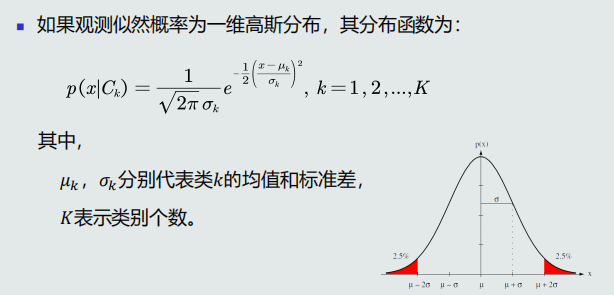• 带入MAP分类器的判别公式：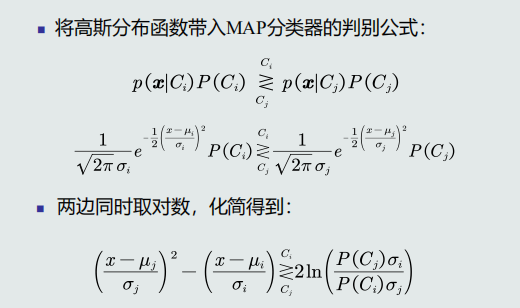• 得到决策边界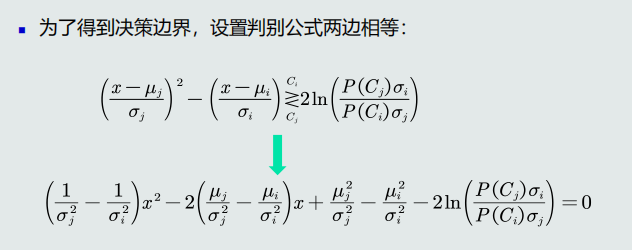• $$𝜎_𝑖=𝜎_𝑗=𝜎$$时，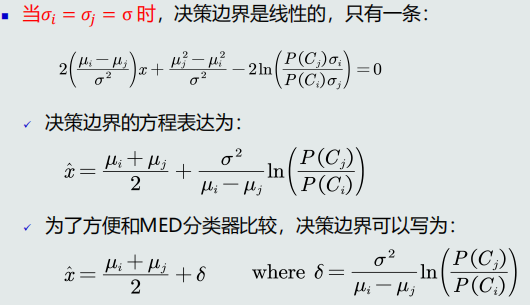• 在方差相同的情况下，MAP决策边界偏向先验可能性较小的类，即分类器决策偏向先验概率高的类。
• $${𝜎_𝑖 }\neq{𝜎_𝑗}$$时，分类器倾向选择方差较小（紧致）的类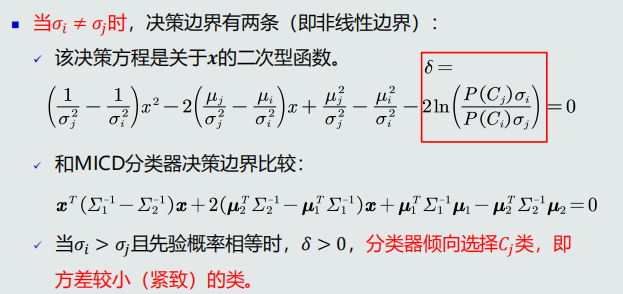• MAP分类器可以解决MICD分类器存在的问题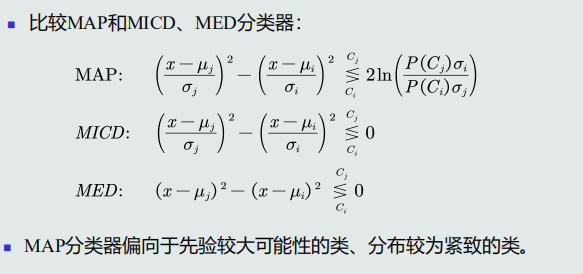• 高维高斯分布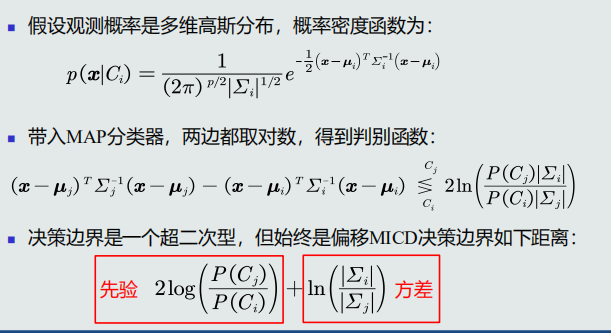#### 决策风险与贝叶斯分类器

• 决策风险：贝叶斯决策不能排除出现错误判断的情况，由此会带来决策风险。
• 损失：定义一个惩罚量，用来表征当前决策动作相对于其他候选类别的风险程度，即损失（loss）。假设该测试样本𝒙 的真值是属于$$𝐶_𝑗$$类，决策动作$$𝛼_𝑖$$对应的损失可以表达为：$$𝜆(𝛼_𝑖|𝐶_𝑗)$$，简写为$$𝜆_𝑖𝑗$$
• 损失的评估：针对所有决策动作和候选类别，可以用一个矩阵来表示对应的损失值
• 决策风险的评估：给定一个测试样本x，分类器决策其属于Ci类的动作αi对应的决策风险可以定义为相对于所有候选类别的期望损失，记作$$R(α_i|x)=\sum_j{λ_{ij}} p(C_j|x)$$
• 判别公式：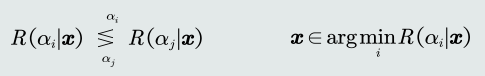• 贝叶斯决策的期望损失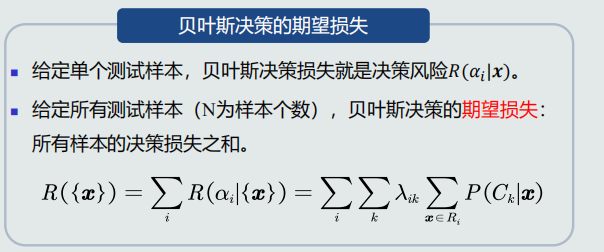• 决策分类器的决策目标
• 给定所有测试样本 {𝒙}，贝叶斯分类器的决策目标：最小化期望损失
• 如何实现期望损失最小化？对每个测试样本选择风险最小的类。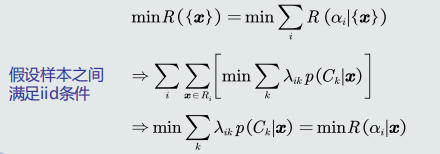• 朴素贝叶斯分类器
• 背景：如果特征是多维，学习特征之间的相关性会很困难。
• 假设特征之间是独立的：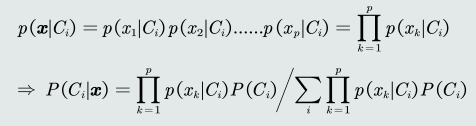• 为了避免出现错误的决策，分类器可以选择拒绝。可以引入阈值τ。当τ=1时所有样本的任何决策都会被拒绝。当τ<1/k时，所有样本的决策都不会被拒绝，k是类别的个数。

#### 最大似然估计

-监督式学习方法：

• 参数化方法：给定概率分布的解析表达，学习这些解析表达函数中的参数。该类方法也称为参数估计。常用的有最大似然估计、贝叶斯估计。
• 非参数化方法：概率密度函数形式未知，基于概率密度估计技术，估计非参数化的概率密度表达。
• 定义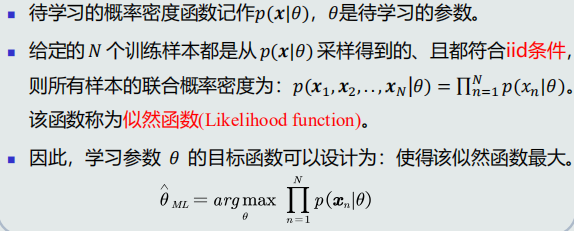• 目标函数：给定所有类的𝑁个训练样本，假设随机抽取其中一个样本属于$$𝐶_1$$类的概率为𝑃，则选取到$$𝑁_1$$个属于$$𝐶_1$$类样本的概率为先验概率的似然函数（即目标函数）。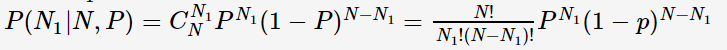其中P是待学习的参数。
• 先验概率估计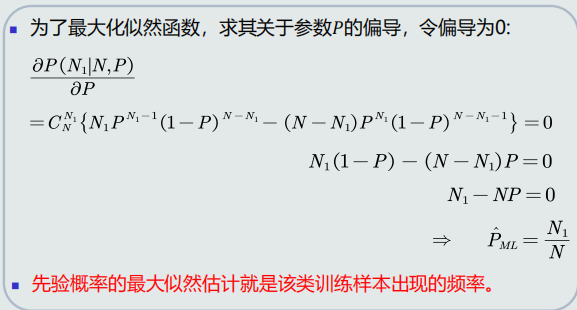• 观测概率估计：高斯分布
• 带学习的参数：如果观测似然概率服从高斯分布，待学习的参数包含该高斯分布的均值𝝁和协方差𝚺。
• 目标函数：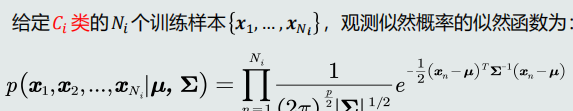• 参数估计：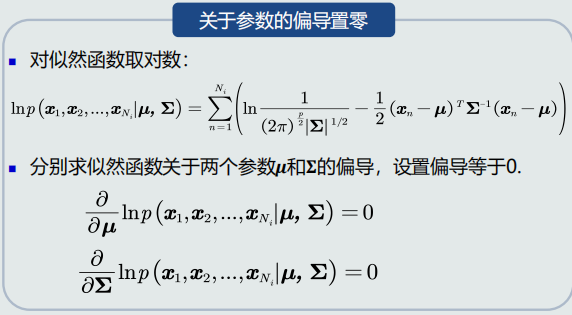• 均值估计：高斯分布均值的最大似然估计等于样本的均值。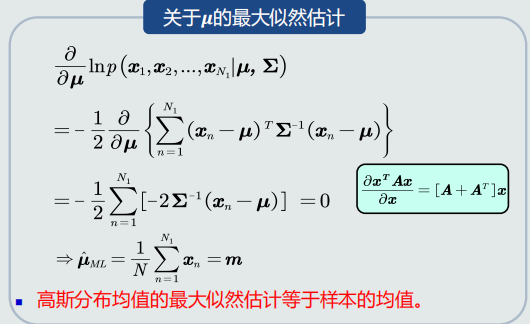• 协方差估计：高斯分布协方差的最大似然估计等于所有训练模式的协方差。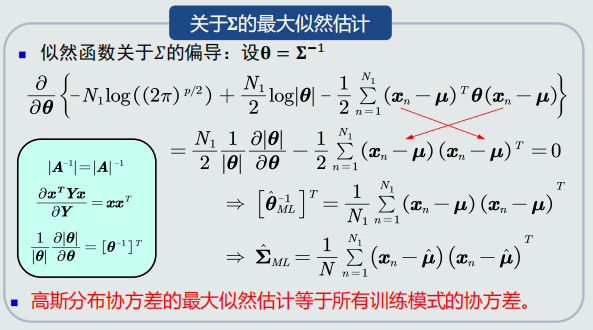#### 最大似然的估计偏差

• 如果一个参数的估计量的数学期望是该参数的真值，则该估计量称作无偏估计。无偏估计意味着只要训练样本个数足够多，该估计值就是参数的真实值。
• 均值的最大似然估计是无偏估计
• 高斯分布协方差的最大似然估计是有偏估计。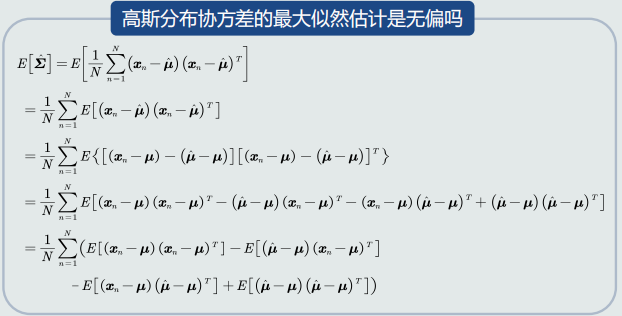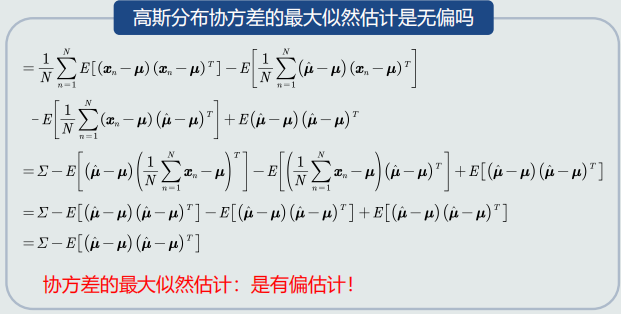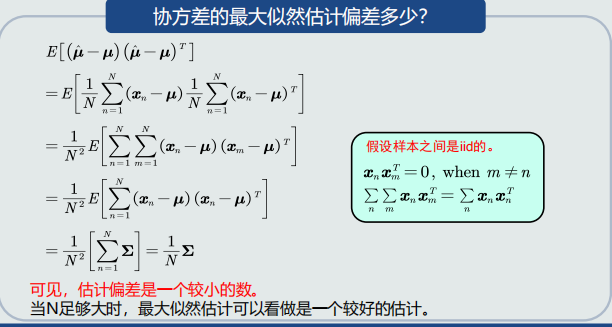• 协方差估计的修正：在实际计算中，可以通过将训练样本的协方差乘以𝑁/(𝑁 − 1)来修正协方差的估计值：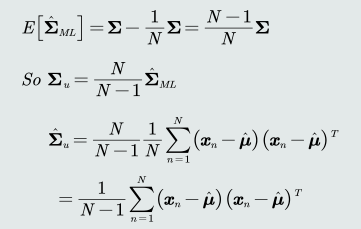#### 贝叶斯估计

• 概率分布中待学习的参数𝜃也可以当做随机变量。
• 贝叶斯估计：给定参数𝜃分布的先验概率以及训练样本，估计参数θ分布的后验概率。
• 假设𝜃服从一个概率分布：
• 该概率分布的先验概率已知：𝑝(𝜃）
• 先验概率反映了关于参数𝜃的最初猜测及其不确定信息
• 后验概率：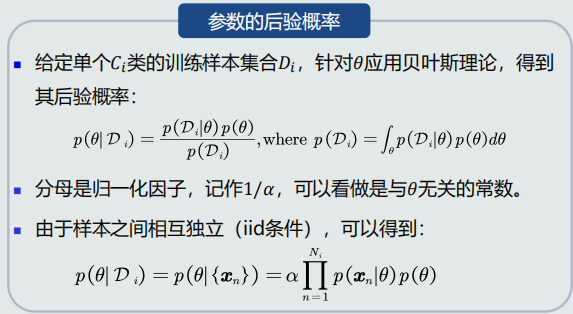• 贝叶斯估计：高斯观测似然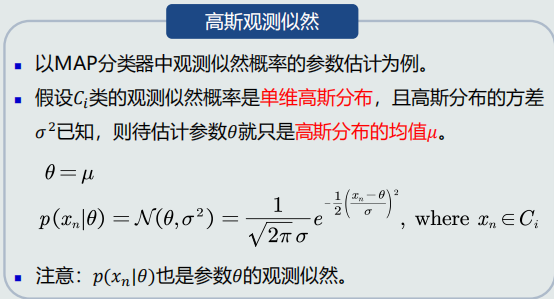• 参数（高斯均值）先验概率：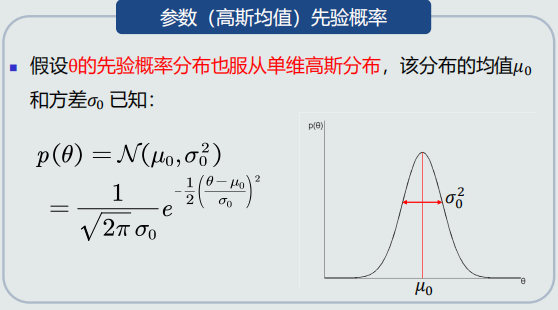• 参数（高斯均值）后验概率：• 给定𝐶𝑖类的𝑁𝑖个训练样本，参数θ概率分布的均值等于训练样本均值和该参数先验概率均值的加权和。
• 给定𝐶𝑖类的𝑁𝑖个训练样本，参数θ概率分布的方差是由𝐶𝑖类观测似然分布的方差、该参数的先验概率方差、𝐶𝑖类的样本个数共同决定。
• 当𝑁𝑖足够大时，样本均值m就是参数θ的无偏估计。
• 参数先验对后验的影响：• 贝叶斯估计：不断学习能力
• 它允许最初的、基于少量训练样本的、不太准的估计。
• 随着训练样本的不断增加，可以串行的不断修正参数的估计值，从而达到该参数的期望真值。
• 贝叶斯估计流程步骤：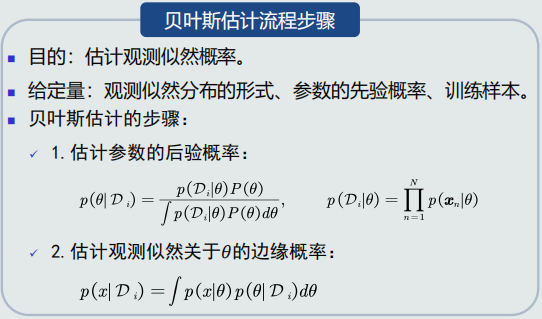#### 无参数概率密度估计

• 常用的无参数技术：K近邻法、直方图技术、核密度估计
• KNN估计：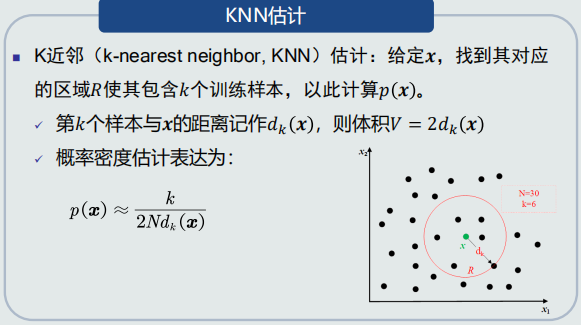• KNN估计优缺点：
• 可以自适应的确定𝒙相关的区域𝑅的范围。
• KNN概率密度估计不是连续函数。
• 不是真正的概率密度表达，概率密度函数积分是 ∞ 而不是1。例如，在k=1时。

#### 直方图与核密度估计

• 直方图也是基于无参数概率密度估计的基本原理：p(x)$$\approx$$k/NV

• 区域R的确定：

• 直接将特征空间分为m个格子(bins)，每个格子即为一个区域𝑅，即区域的位置固定。
• 平均分格子大小，所以每个格子的体积（带宽）设为𝑉 = ℎ，即区域的大小固定。
• 相邻格子不重叠。
• 落到每个格子里的训练样本个数不固定，即𝑘值不需要给定
• 优缺点：

• 固定区域𝑅：减少由于噪声污染造成的估计误差。不需要存储训练样本。
• 固定区域𝑅的位置：如果模式𝒙落在相邻格子的交界区域，意味着当前格子不是以模式𝒙为中心，导致统计和概率估计不准确。
• 固定区域𝑅的大小：缺乏概率估计的自适应能力，导致过于尖锐或平滑
• 双线性插值：针对区域R的位置固定，自适应能力不强的问题

• 带宽选择：带宽ℎ过小，概率密度函数过于尖锐。带宽ℎ过大，概率密度函数过于平滑。

• 核密度估计

• 区域R的确定：以任意待估计模式𝒙为中心、固定带宽ℎ，以此确定一个区域𝑅。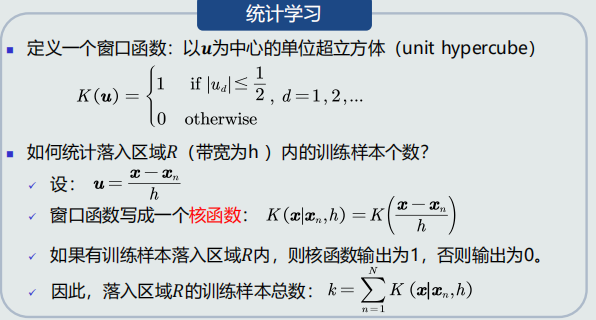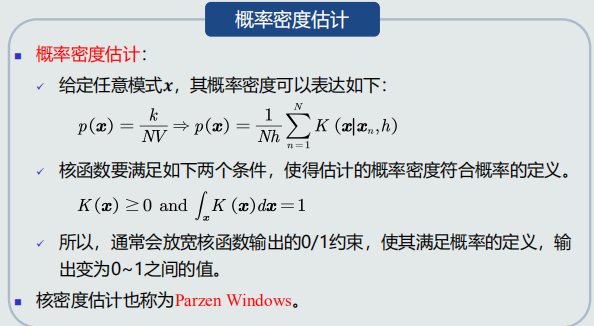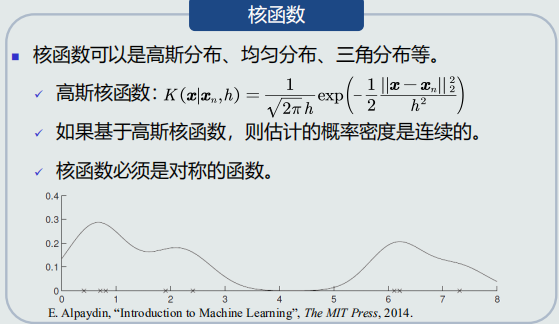• 优缺点：
• 以待估计模式𝒙为中心、自适应确定区域𝑅的位置（类似KNN）。
• 使用所有训练样本，而不是基于第 𝑘 个近邻点来估计概率密度，从而克服KNN估计存在的噪声影响。
• 如果核函数是连续，则估计的概率密度函数也是连续的。
• 与直方图估计相比，核密度估计不提前根据训练样本估计每个格子的统计值，所以它必须要存储所有训练样本。
• 带宽选取原则：泛化能力
• 带宽ℎ决定了估计概率的平滑程度。
• 因为给定的训练样本数量是有限的，所以要求根据这些训练样本估计出来的概率分布既能够符合这些训练样本，同时也要有一定预测能力，即也能估计未看见的模式。

### 线性判据与回归

#### 线性判据

• 生成模型：给定训练样本 {$$𝒙_𝑛$$}，直接在输入空间内学习其概率密度函数𝑝(𝒙)
• 在贝叶斯决策分类中，生成模型通常用于估计每个类别的观测似然概率 $$𝑝(𝒙|𝐶_𝑖)$$,再结合先验概率得到联合概率$$𝑝(𝒙,𝐶_𝑖)=𝑝(𝒙|𝐶_𝑖)𝑝(𝐶_𝑖)$$ 。然后，对所有类别进行积分，得到边缘概率密度函数$$𝑝(𝒙)=\sum_i𝑝(𝒙,𝐶_𝑖)$$。最后，得到后验概率$$𝑝(𝐶_𝑖|𝒙)$$$$p(C_i|x)=\frac{p(x|C_i)p(C_i)}{p(x)}$$
• 优势：可以根据𝑝(𝒙)采样新的样本数据；可以检测出较低概率的数据，实现离群点检测
• 劣势：如果是高维的𝒙，需要大量训练样本才能准确的估计𝑝(𝒙) ；否则，会出现维度灾难问题。
• 判别模型：
• 给定训练样本$${𝒙_𝑛}$$，直接在输入空间内估计后验概率$$𝑝(𝐶_𝑖|𝒙)$$
• 在分类任务中，后验概率分布$$𝑝(𝐶_𝑖|𝒙)$$相当于直接从输入样本𝒙映射
到类别输出$$𝐶_𝑖$$的判别函数𝑓(𝒙)，即判别模型。
• 优势：快速直接、省去了耗时的高维观测似然概率估计。（在分类任务中，观测概率分布所包含的大量复杂内容有时对于后验概率的影响比较有限）

#### 最简单的判别模型

• 线性判据：如果判别模型𝑓(𝒙)是线性函数，则𝑓(𝒙)为线性判据。
• 可以用于两类分类，决策边界是线性的。
• 也可以用于多类分类，相邻两类之间的决策边界也是线性的。
• 计算量少：在学习和分类过程中，线性判据方法都比基于学习概率分布的方法计算量少。
• 适用于训练样本较少的情况。
• 线性判据：数学表达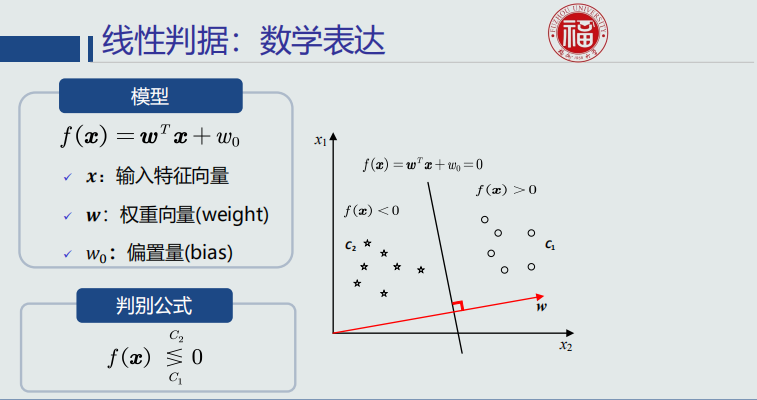• 线性判据：决策边界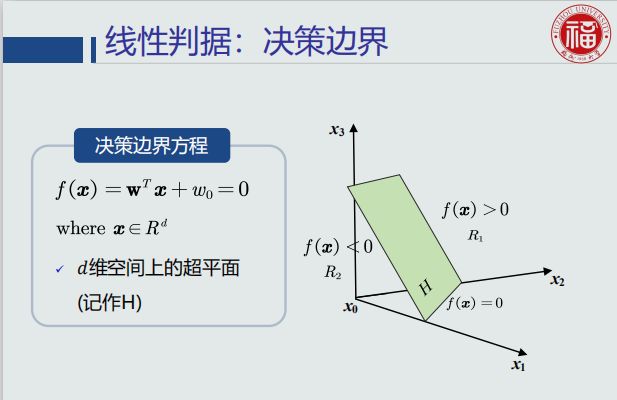• 𝒘的作用：𝒘垂直于决策边界上的任何向量，即𝒘垂直于决策边界H，是H的法向量。决定了决策边界H的方向。
• 线性判据：$$W_0$$的作用：$$W_0$$决定了决策边界的偏移量，使其能够满足两个类输出值分别为正负。
• 任意样本到决策边界的距离，该距离𝑟的绝对值可以作为confidence score：值越大，这个点属于正类或者负类的程度越大。 𝑓(𝒙)是样本𝒙到决策面𝐻的代数距离度量。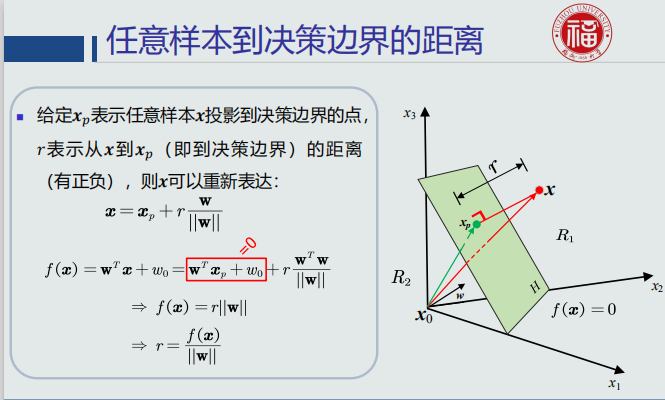• 𝑤0决定决策边界相对于坐标原点的位置。
• 线性判据：学习和识别过程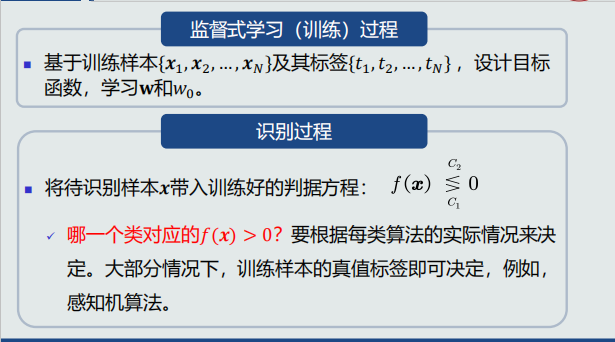• 解不唯一， 解域：在参数空间内，参数的所有可能解所处的范围。
-找到最优解——目标函数的求解：最小化/最大化目标函数。
• 涉及优化技术。
• 解析求解：求关于训练参数的偏导，并设置偏导为0.
• 迭代求解：先猜测参数初始值，然后不断的根据当前计算得到的更新值迭代更新参数。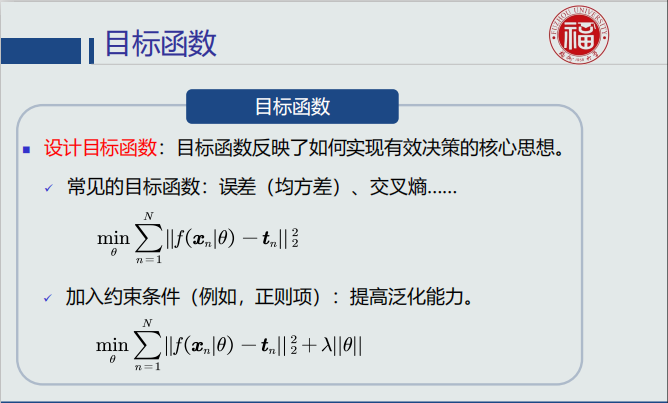• 加入约束条件：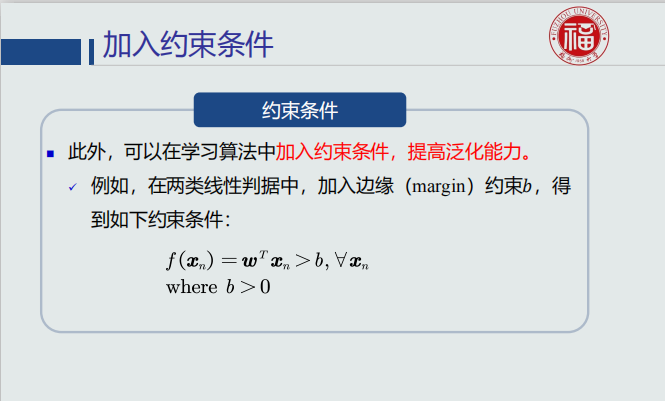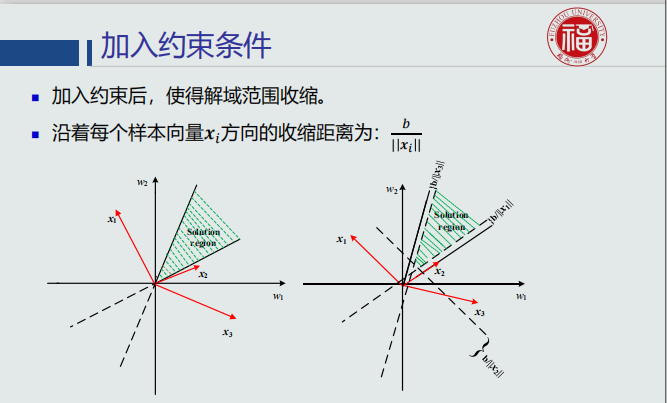#### 并性感知机算法

• 预处理：将两个参数合为一个参数𝒂，将𝐶2类的训练样本全部取反（预处理后两个类的输出值都为正数。）
• 几何解释：在几何上，通过在特征空间上增加一个维度，使得决策边界可以通过原点（𝑤0项）。翻转𝐶2类的样本：得到一个平面使得所有样本位于该平面同一侧。
• 目标函数：：针对所有被错误分类的训练样本（即输出值小于0的训练样本），其输出值取反求和：$$J(a)=-\sum_{y∈Y}a^T𝒚,𝒀 = 𝒚|𝒂^𝑇𝒚 ≤ 0$$,该目标函数是关于a的一次线性函数。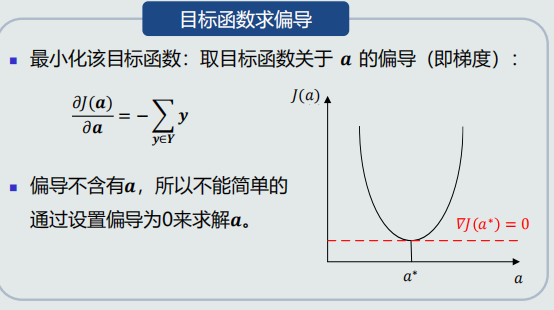• 梯度下降法：
• 使用当前梯度值迭代更新参数。更新的方向（正负）：假设在k=1迭代时刻，参数取值$$𝒂_1$$情况下，每个维度的梯度反方向就是该维度往目标函数最小值收敛的最速下降方向。
• 更新的大小：每个维度的梯度幅值代表参数在该维度上的更新程度。通常加入步长(𝜂𝑘)来调整更新的幅度。每次迭代可以用不同的步长。
• 参数更新：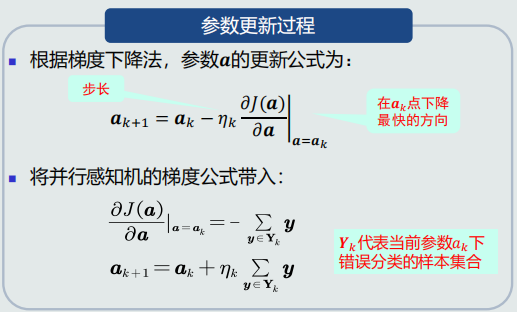• 算法流程：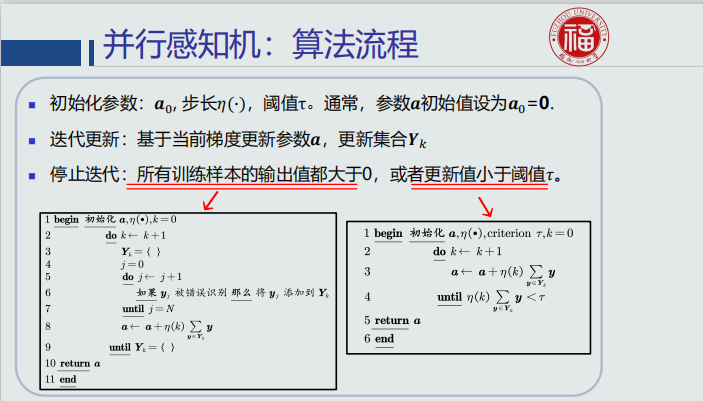#### 串行感知机算法

• 训练样本是一个一个串行给出的。
• 目标函数：如果当前训练样本被错误分类，最小化其输出值取反：$$J(a)=-a^Ty_n,if a^Ty_n≤ 0$$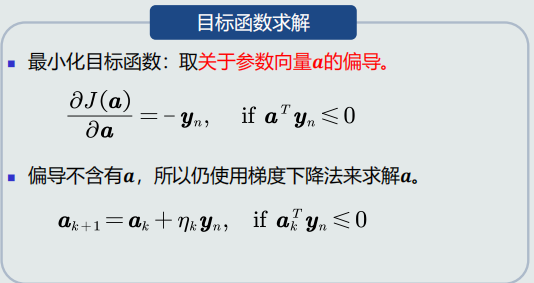• 算法流程：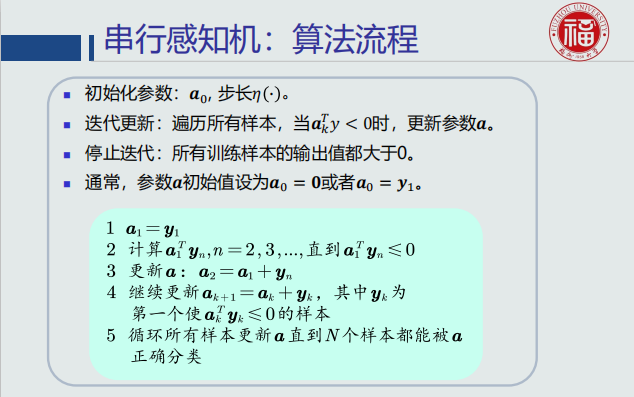• 收敛性：：如果训练样本是线性可分的，感知机（并行和串行）算法理论上收敛于一个解。
• 步长决定收敛的速度、以及是否收敛到局部或者全局最优点。
• 如果目标函数J(a)满足L-Lipschitz条件（对于任意a，存在一个常数L，使得|J(a)|<L成立），则步长𝜂 = 1/ 2𝐿 可确保收敛到局部最优点。
• 如果目标函数J(a)是凸函数，局部最优点就是全局最优点。
• 提升感知机的泛化能力：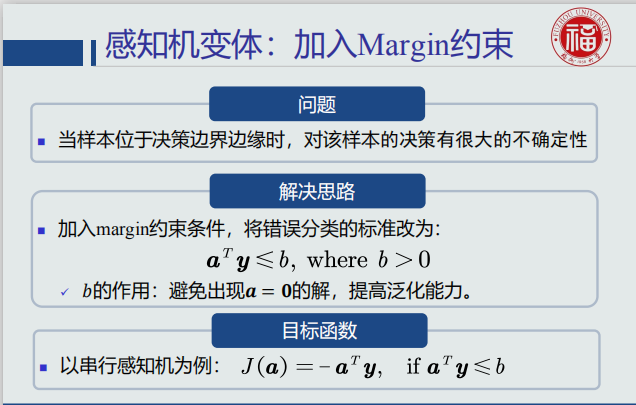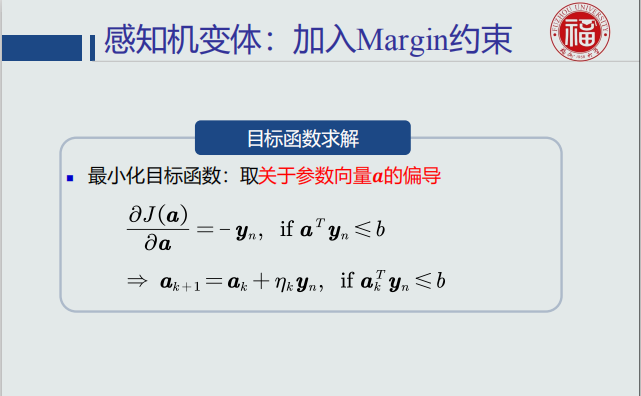#### Fisher线性判据

• 基本原理：找到一个最合适的投影轴，使两类样本在该轴上投影的重叠部分最少，从而使分类效果达到最佳。
• 最佳标准之一：投影后，使得不同类别样本分布的类间差异尽可能大，同时使得各自类内样本分布的离散程度尽可能小。
• 表征量的数学表达：
• 类间样本的差异程度：用两类样本分布的均值之差度量。
• 类内样本的离散程度：用每类样本分布的协方差矩阵表征。
• 目标函数：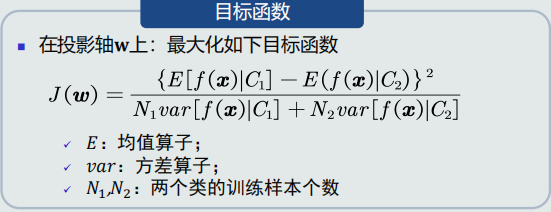• 目标函数优化：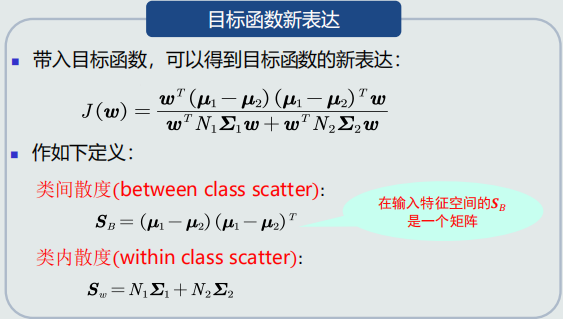• 求解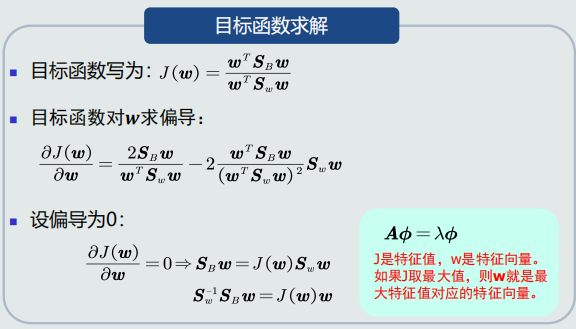• 最优参数解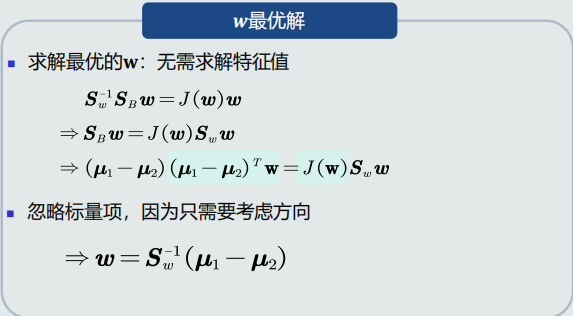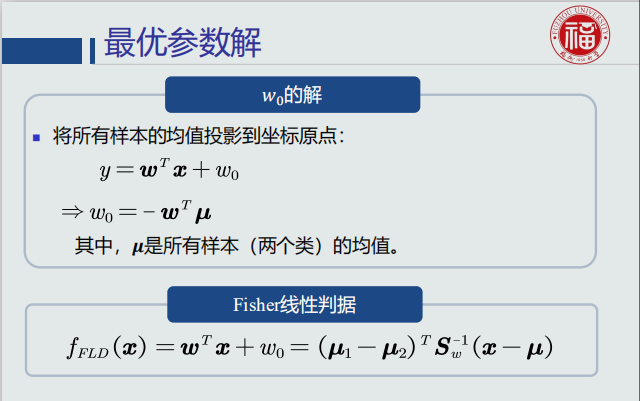• 决策边界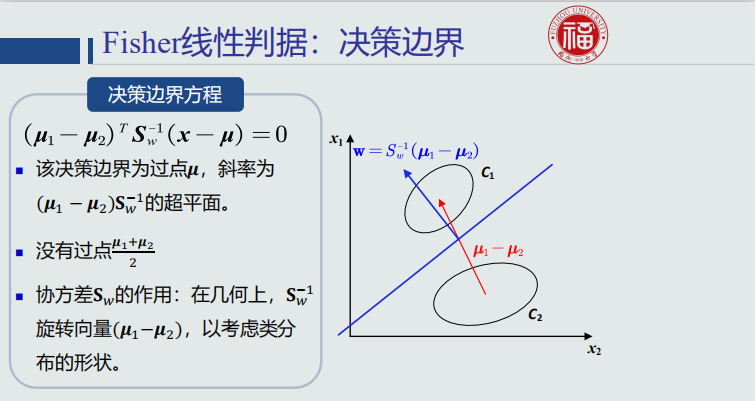#### 支持向量机

• 设计思想： 给定一组训练样本，使得两个类中与决策边界最近的训练样本到决策边界之间的间隔最大。
• 间隔：在两个类的训练样本中，分别找到与决策边界最近的两个训练样本，记作𝒙+和 𝒙− 。𝒙+和𝒙−到决策边界的垂直距离叫作间隔，记作𝑑+和𝑑− 。
• 支持向量在确定决策边界中起到核心作用。
• 间隔计算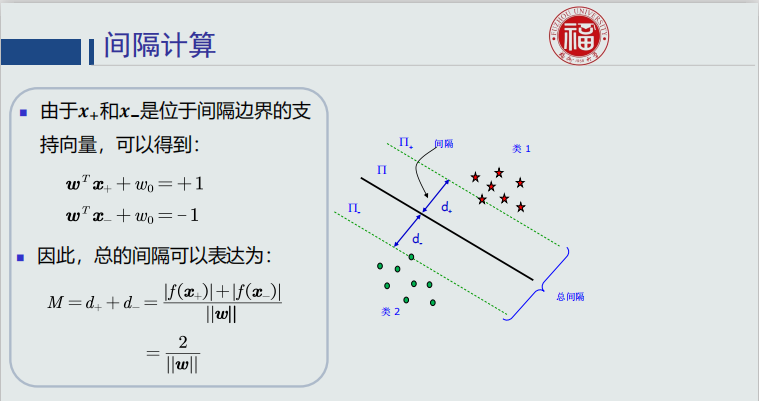• 目标函数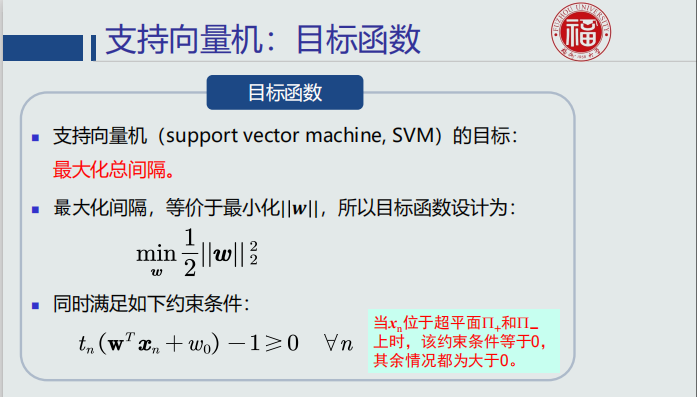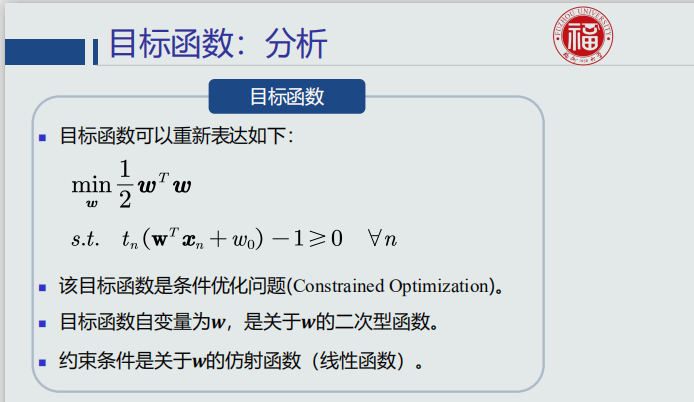#### 拉格朗日乘数法

• 支持向量机的目标函数是一个条件优化问题，拉格朗日乘数可以解决该问题。
• 条件优化问题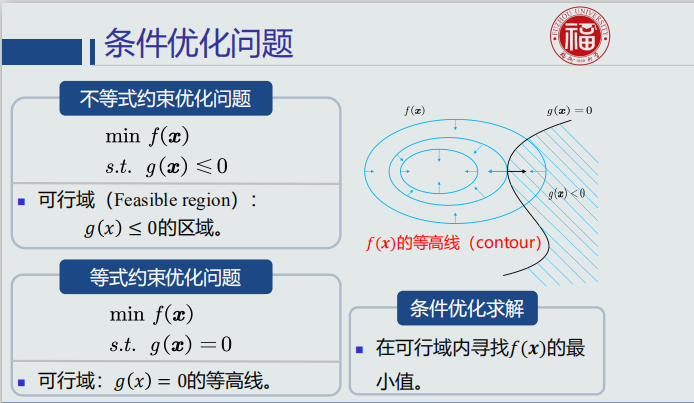• 等式约束
• 函数在等高面上任意一点的梯度方向与其等高面（切线方向）正交，且朝向（即正方向）函数值较高的方向。
• 𝑓(𝒙)的极值点𝒙∗必须位于曲线𝑔(𝒙)=0上。沿着𝑔(𝒙)= 0的切线方向、向着𝑓(𝒙)负梯度方向移动。当出现沿着切线方向、无法再向𝑓(𝒙)负梯度方向移动时停止.
• 𝑓(𝒙)负梯度方向，即梯度下降最快方向,𝑓(𝒙)取值沿着该方向不断减小。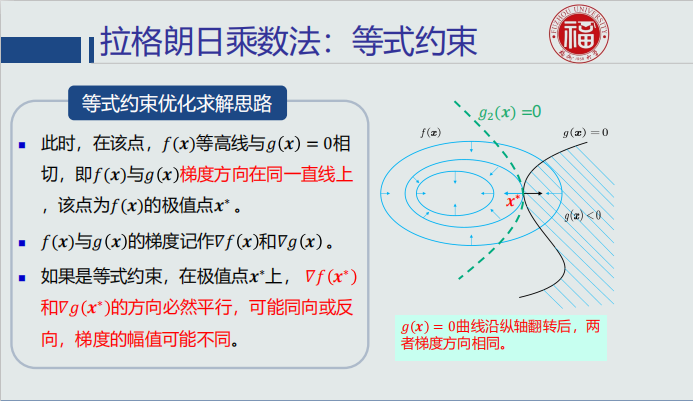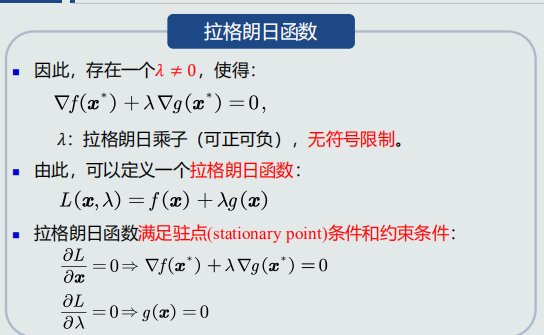• 拉格朗日乘数法：不等式约束
• 情况1：极值点落在可行域内,直接通过𝛻𝑓(𝒙)= 0获得极值点。
• 情况2：极值点落在可行域边界,g(x)=0,搜寻极值点𝒙∗：当出现沿着g(x)= 0切线方向、无法再向𝑓(𝒙)负梯度方向移动时停止。在该点,𝑓(𝒙)等高线与g(x)= 0相切，该点为𝑓(𝒙)的极值点𝒙∗ 。对于不等式约束，在极值点𝒙∗，𝑓(𝒙)与g(x)的负梯度方向平行且相反。梯度的幅值可能不同。
• 综合两种情况：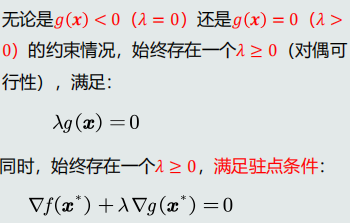• 不等式约束——KKT条件：在𝑔(X)≤ 0约束条件下最小化𝑓(𝒙)的问题，可以转化为如下约束条件
（KKT条件）下的拉格朗日函数优化问题：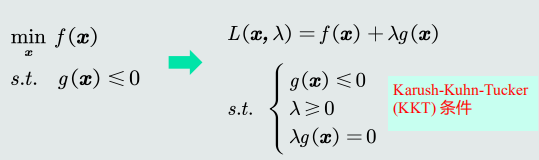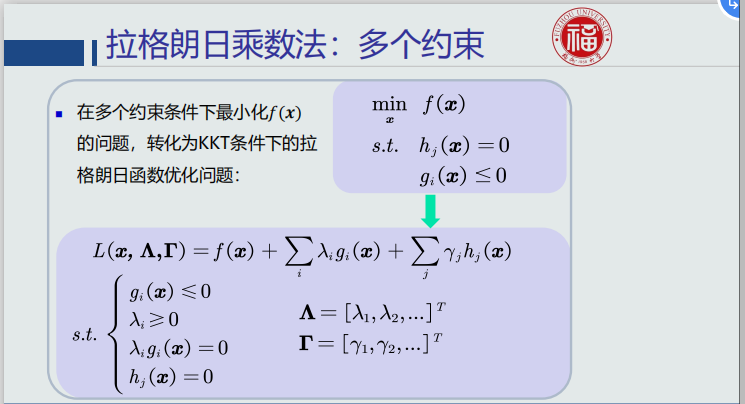#### 拉格朗日对偶问题

• 拉格朗日对偶函数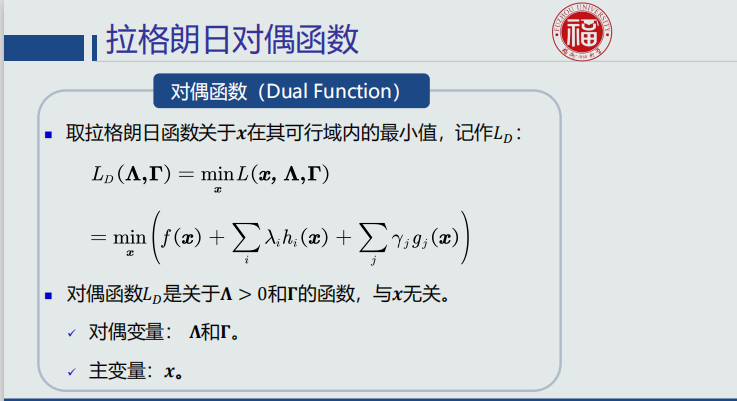• 主问题最优值的下界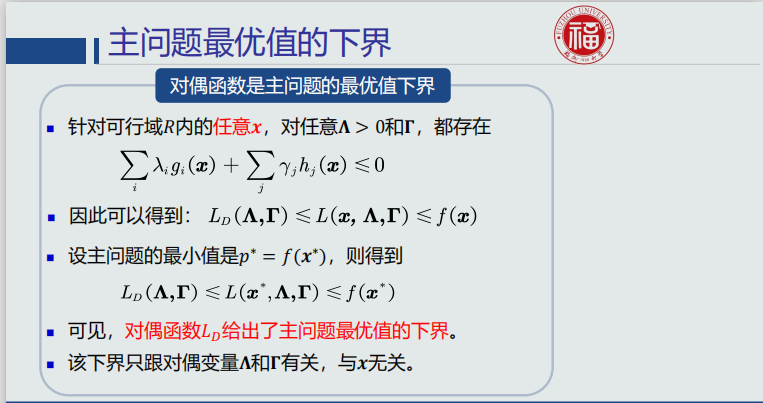• 对偶问题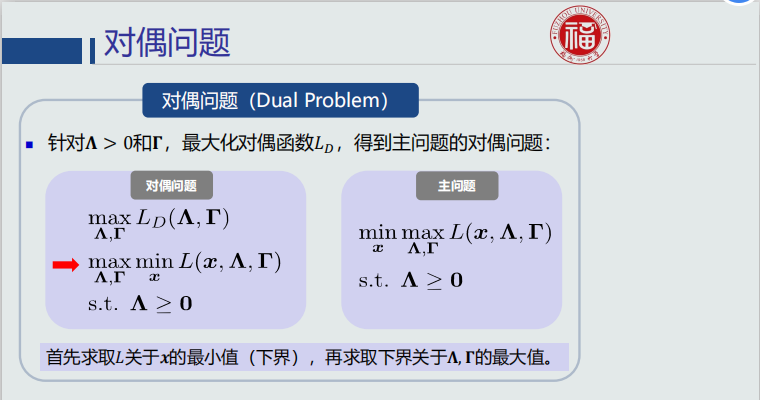• 对偶函数凹凸性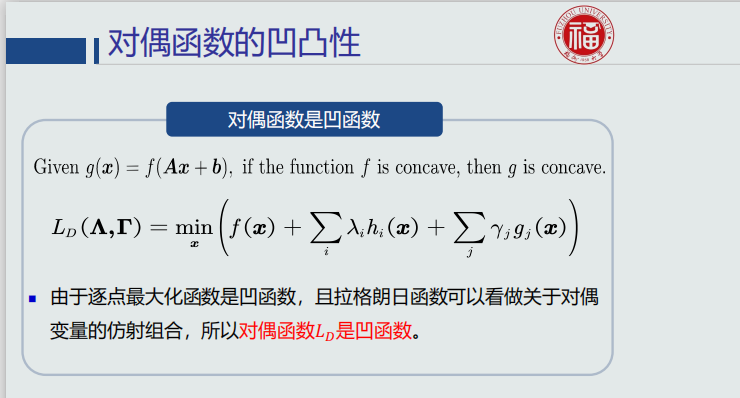-对偶法优势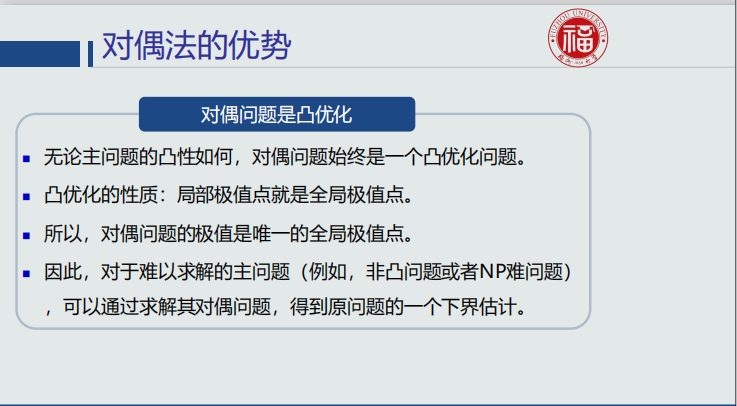• 弱对偶性
• 设对偶问题的最优值为𝑑∗、主问题的最优值为𝑝∗。
• 对于所有的优化问题都存在：𝑑∗≤𝑝∗
• 强对偶性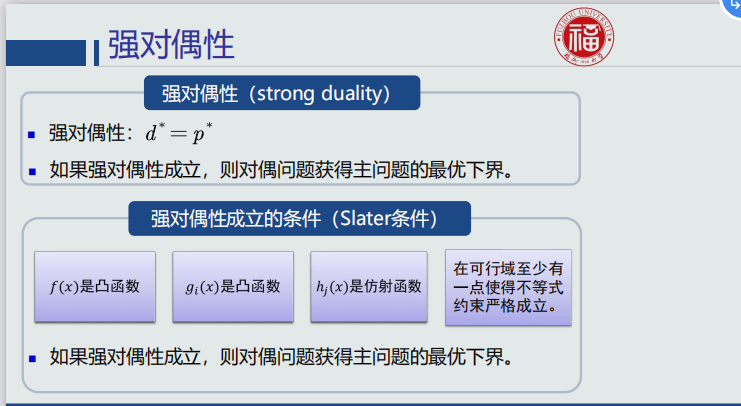#### 支持向量机学习算法

• 构建拉格朗日函数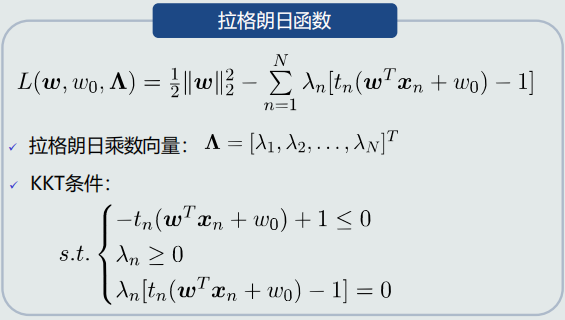• 构建对偶函数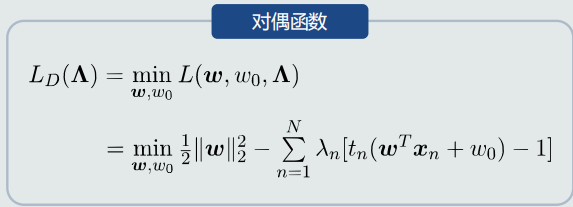• 对偶函数的约束条件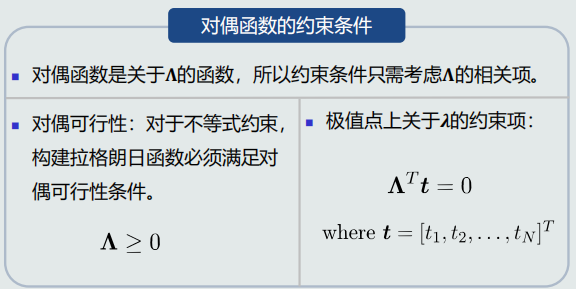• 对偶问题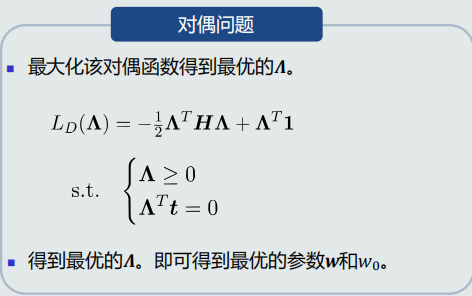• 对偶问题求解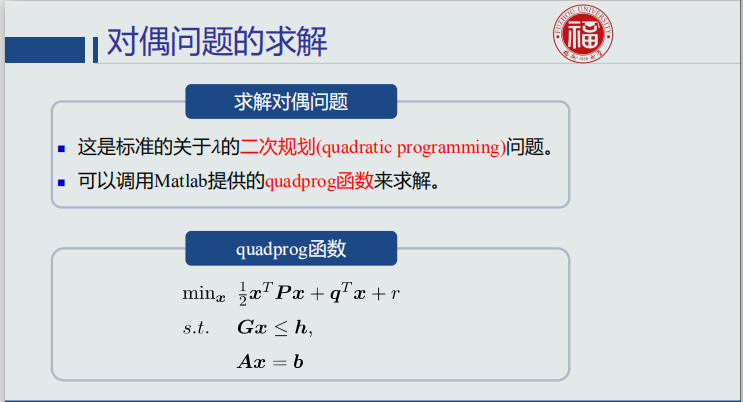• 求解支持向量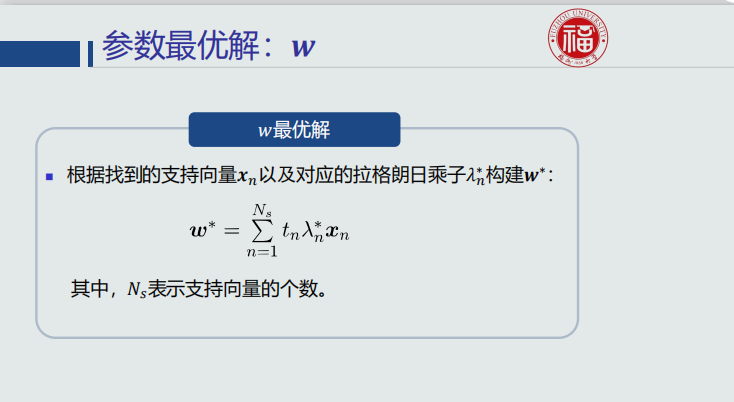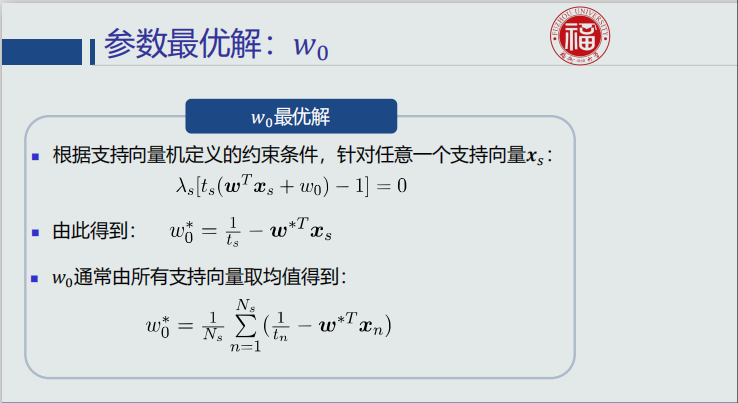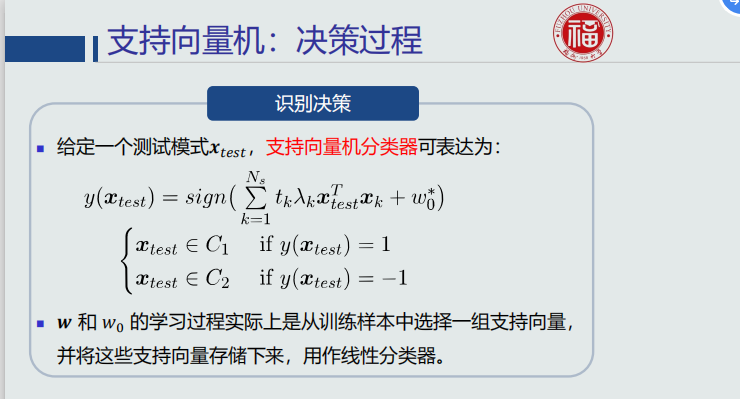posted @ 2020-05-06 00:39  陈秋琴  阅读(518)  评论(0编辑  收藏  举报